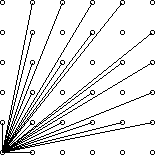# 欧拉函数研究札记

## 数学的世界

Posted by     LYC on May 20, 2018

PJQOOO的13级ACM暑假集训

Kevin_naticl’s欧拉函数讲解（where the product is over the distinct prime numbers dividing n. 基础英语我就不翻译了

• φ(1)=1（和1互质的数(小于等于1)就是1本身）。 注意：每种质因数只一个。 比如12=223那么φ（12）=12（1-1/2）(1-1/3)=4

• 若n是质数p的k次幂，那么有：• 重要：欧拉函数是积性函数(Multiplicative function)——若m,n互质，那么有：• 重要：若n为质数则有：The first thousand values of φ(n). The points on the top line represent φ(p) when p is a prime number, which is p−1

## 欧拉函数表达式的推导

• The function is multiplicative.（这个函数是积性函数）

• Value for a prime power argument.（where the product is over the distinct prime numbers dividing n.）

## 应用

SDOI2008仪仗队### 求欧拉函数的代码

``````int ola(int n)
{
int ans=0,i,k;
if(n==1)
ans=1;
else
{
ans=n;
k=1;
for(i=2;n!=1;i+=k)
{
if(n%i==0)
{
ans/=i;
ans*=(i-1);
while(n%i==0) n/=i;
i=k;
}
}
}
return ans;
}
``````

END